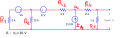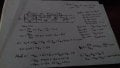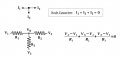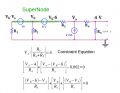# Finding voltage source on circuit

#### csstudent

Joined Oct 22, 2019
15
Hello, I've been having difficulty dealting with circuit analysis.
Here, for example, I'm given the task to find the voltage on Vs. But I fail short half-way through.
Also, I don't understand how to deal with negative voltages and/or currents, and would appreciate help!My attempt at solving this:
V(R5)=4 V because parallel to that thing
I(R4)=4V/1k=4mA=I(R3)
2k=V(R4)/4mA => V(R4)=2k*4m=8 V

Then I applied mesh equation to last mesh:
V(IA)+8V+4V=0 <=> V(IA)=-12 V (How?)

Node equation at a:
2mA=4mA+I(R3) => I(R3)=-2mA (What does this mean?)

V(R3)=3k*(-2mA)=-6 V

Mesh on middle:
-12V-(-6V)-(-6V)+V(R2)=0
V(R2)=0 Impossible

Please help me understand what I did wrong, and consider as if I was very dumb (which is probably true).

Last edited:

#### WBahn

Joined Mar 31, 2012
26,139
Hello, I've been having difficulty dealting with circuit analysis.
Here, for example, I'm given the task to find the voltage on Vs. But I fail short half-way through.
Also, I don't understand how to deal with negative voltages and/or currents, and would appreciate help!
View attachment 189464
My attempt at solving this:
V(R5)=4 V because parallel to that thing
I(R4)=4V/1k=4mA=I(R3)
2k=V(R4)/4mA => V(R4)=2k*4m=8 V

Then I applied mesh equation to last mesh:
V(IA)+8V+4V=0 <=> V(IA)=-12 V (How?)

Node equation at a:
2mA=4mA+I(R3) => I(R3)=-2mA (What does this mean?)

V(R3)=3k*(-2mA)=-6 V

Mesh on middle:
-12V-(-6V)-(-6V)+V(R2)=0
V(R2)=0 Impossible

Please help me understand what I did wrong, and consider as if I was very dumb (which is probably true).
One thing you need to be careful about is making sure that you define your polarities. When you say V(R2) (the voltage across R2), what is the polarity? You haven't defined it. This WILL lead you into problems sooner or later (most probably sooner) as you mess up the signs in your work.

In your second line of work you have

I(R4)=4V/1k=4mA=I(R3)

Again, what direction is I(R3) and I(R4)? There's no way for anyone to know.

Assuming they are both left to right, then how can they be equal given that you have 2 mA flowing into Node a?

As for negative and positive currents and voltages, the reason that you are confused is probably directly related to the failure to define the polarities. If I define the polarity of a current as flowing from left to right through a component, then if that current turns out to be negative, it simply means that the current is really flowing right to left.

#### csstudent

Joined Oct 22, 2019
15
One thing you need to be careful about is making sure that you define your polarities. When you say V(R2) (the voltage across R2), what is the polarity? You haven't defined it. This WILL lead you into problems sooner or later (most probably sooner) as you mess up the signs in your work.

In your second line of work you have

I(R4)=4V/1k=4mA=I(R3)

Again, what direction is I(R3) and I(R4)? There's no way for anyone to know.

Assuming they are both left to right, then how can they be equal given that you have 2 mA flowing into Node a?

As for negative and positive currents and voltages, the reason that you are confused is probably directly related to the failure to define the polarities. If I define the polarity of a current as flowing from left to right through a component, then if that current turns out to be negative, it simply means that the current is really flowing right to left.
Thank you, friend. Words can not express my gratitude!
It really was a question of defining directions from the get-go. Sorry for the newbish question but: is the directions I set at the beggining irrelevant? Like, if I had set some of them in opposite directions, would I still get the same result?
Also, here's how I did it. If you have any tips for improvement, please feel free to share!#### WBahn

Joined Mar 31, 2012
26,139
The directions you choose don't affect the result. If you pick a current direction in one direction and get a positive number for your answer, then had you picked the opposite direction for that current you would have gotten a negative number for your answer -- but these are the SAME result.

Because humans are much more prone to make silly errors when working with negative numbers, if you assign your directions such that you expect the results to be positive, then you can avoid some of these. Also, if you get a negative result you have a red flag that you can look at more closely to either catch a mistake or gain a better understanding of what is going on by confirming that the negative result is actually correct.

Having said that, for the more formal analysis methods, such as node voltage and mesh current, it is useful to adopt a consist way of defining your polarities and then stick to it even if you can tell that some of them will come out to be negative. The advantages of applying a well-practiced analysis technique consistently usually outweighs the benefit of avoiding negative answers.

#### RBR1317

Joined Nov 13, 2010
562
This circuit seems like a good candidate for nodal analysis. When designing and working with small-signal circuits one is generally more interested in measuring voltages with respect to a common ground, i.e. node voltages (which I believe are conceptually simpler than circulating mesh currents.) A node equation expresses the notion that the sum of all currents flowing from a node must equal zero. Assume that all analytical currents flow from the node and are positive; defined currents from a current source will be negative if flowing into the node. Analytical currents are expressed in voltages in the form: (Vnode - Vadjacent)/(Resistance between nodes). There is no need to worry about polarity except for the direction of defined currents. Also, every term in a node equation will begin with the voltage of that node, making it easier to check the correctness of the node equation. Being consistent is the path to avoid mistakes.So how are these simple rules for writing node equations applied in the given circuit which contains a supernode (a node containing defined voltage offsets)? I find it useful to highlight the extent of the supernode and mark the voltage offsets on the schematic for writing the supernode equation. If there is more than one supernode then use different highlight colors. For this problem a constraint equation is necessary in addition to the two node equations (there are three unknown voltages: 2 node voltages & 1 source voltage, so 3 equations are needed for a solution).Finally, solving the node equations in Maple. Vs = 36V